Next: Recursive calls to compute Up: Limits within [-1,1] Previous: Input digits required

### A recursive definition

We now show how one might use the approach described above to give a recursive definition for this algorithm. The approach is as follows; we use an auxiliary function f' to do the work hard work, and call it with the correct parameters by the function f, which is the function we ultimately want to use. The function f' takes a parameter sig which determines the number of digits examined since the last digit was decided (or rather the amount by which the further digits examined can modify the range of the finite number of digits examined at the head of x and z so far). Two parameters r and s determine the range of the lower and upper bounds given the first few digits of x and z seen so far, all multiplied by eight. Parameters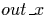and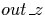are used to store the digits already seen in streams x and z.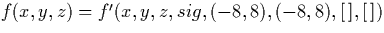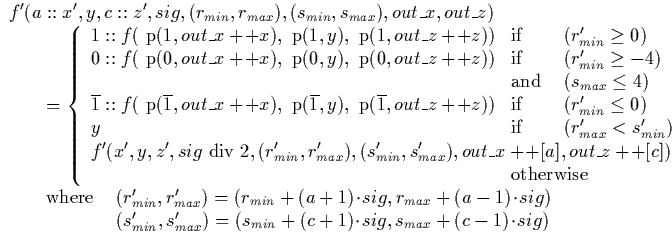In this definition, the function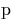is the `p' function defined in section 4.1.4, and a double plus (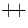) is used to denote concatentation of two lists.

The implementation of this function in Haskell is given in appendix B.Next: Recursive calls to compute Up: Limits within [-1,1] Previous: Input digits required
Martin Escardo
5/11/2000